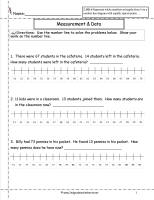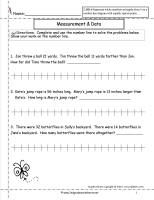﻿ CCSS 2.MD.6 Worksheets, Number Line Data Worksheets

# Common Core State Standard 2.MD.6

## Measurement & Data

### Relate addition and subtraction to length.

Represent whole numbers as lengths from 0 on a number line diagram with equally spaced points corresponding to the numbers 0, 1, 2, …, and represent whole-number sums and differences within 100 on a number line diagram.

###Number Line Data

Common Core State Standards: 2.MD.6
Measurement & Data
Relate addition and subtraction to length.

Represent whole numbers as lengths from 0 on a number line diagram with equally spaced points corresponding to the numbers 0, 1, 2, …, and represent whole-number sums and differences within 100 on a number line diagram.

###Number Line Data

Common Core State Standards: 2.MD.6
Measurement & Data
Relate addition and subtraction to length.

Represent whole numbers as lengths from 0 on a number line diagram with equally spaced points corresponding to the numbers 0, 1, 2, …, and represent whole-number sums and differences within 100 on a number line diagram.